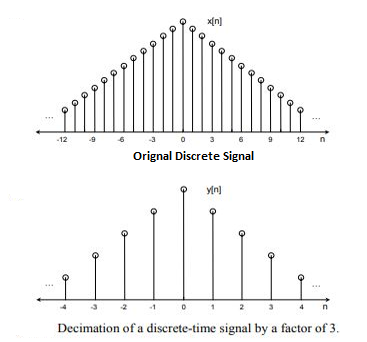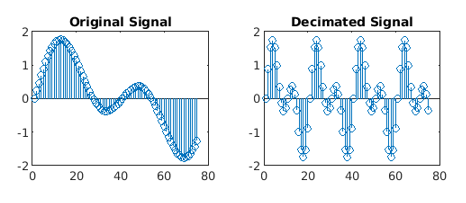Related Articles

# Down-sampling in MATLAB

• Last Updated : 01 Oct, 2020

The two basic operations in a multi-rate system are decreasing/down-sampling (decimation) and increasing (interpolation) the sampling rate of a signal.

In down-sampling we start with a constant time signal x(t) and convert it into a succession of tests x[n], in decimation we start with a discrete-time signal x[n] and convert it into another discrete-time signal y[n], which comprises of sub-tests of x[n].We will be using the decimate() and stem() function.
The decimate() function is used to decrease a sample rate by an integer factor.

Syntax: a = decimate(x, r)
Parameters:

• x: input signal,
• r: decimation factor

Return Value: Decimated Signal

The stem() function is used to plot a discrete sequence data.

Syntax: stem(y)
Parameter:

• y: data sequence

Return Value: Plot of data sequence in discrete time

MATLAB code for down-sampling:

 `% Time vector``t = 0 : .00025 : 1;`` ` `% Original signal``x = sin(2 * pi * 50 * t) + sin(2 * pi * 100 * t);`` ` `% Reduces the sample rate of original signal by factor of 4``y = decimate(x, 4); `` ` `figure()``subplot(2, 2, 1);`` ` `% Plot few samples of the Original signal``stem(x(1:75)) ``title(``'Original Signal'``);`` ` `subplot(2, 2, 2);`` ` `% Plots few samples of the Decimated signal``stem(y(1:75)); ``title(``'Decimated Signal'``);`

OutputMy Personal Notes arrow_drop_up